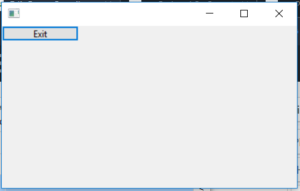# wxPython | Exit() function in wxPython

• Last Updated : 15 Jun, 2020

In this article we are going to learn about wx.Exit() which is a inbuilt parent function present in wxPython.Exit() function exits application after calling wx.App.OnExit .

Should only be used in an emergency: normally the top-level frame should be deleted (after deleting all other frames) to terminate the application. See wx.CloseEvent and wx.App.

Syntax:
wx.Exit()

Parameters:
No parameters are required by Exit() function

Coding Example:

 `import` `wx`` ` ` ` `class` `Example(wx.Frame):`` ` `    ``def` `__init__(``self``, ``*``args, ``*``*``kwargs):``        ``super``(Example, ``self``).__init__(``*``args, ``*``*``kwargs)`` ` `        ``self``.InitUI()`` ` `    ``def` `InitUI(``self``):`` ` `        ``self``.locale ``=` `wx.Locale(wx.LANGUAGE_ENGLISH)``        ``self``.panel ``=` `wx.Panel(``self``, pos ``=``(``100``, ``100``), size ``=``(``100``, ``100``))``        ``self``.btn ``=` `wx.Button(``self``.panel, ``id` `=` `2``, label ``=``"Exit"``, pos ``=` `wx.DefaultPosition, size ``=``(``100``, ``20``))`` ` `        ``self``.Bind(wx.EVT_BUTTON, ``self``.onclick, ``self``.btn)`` ` `    ``def` `onclick(``self``, e):``        ``# EXITS APPLICATION ON CLICKING EXIT BUTTON``        ``wx.Exit()`` ` `def` `main():``    ``app ``=` `wx.App()``    ``ex ``=` `Example(``None``)``    ``ex.Show()``    ``app.MainLoop()`` ` ` ` `if` `__name__ ``=``=` `'__main__'``:``    ``main()`

Output Window:My Personal Notes arrow_drop_up